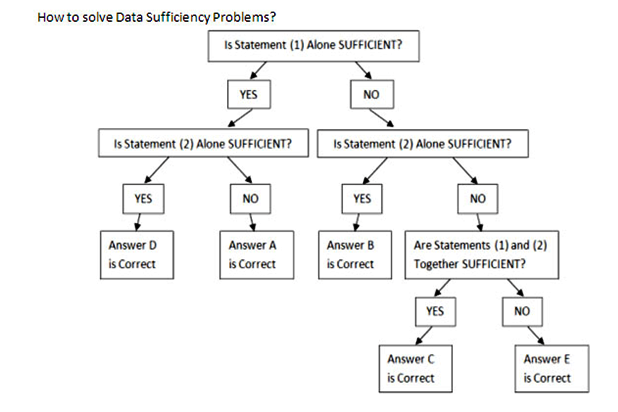Our Address: FZ LLC G 37 & G 34, Block 13, Al Sufouh Road Knowledge Park, Dubai PO Box : 501703

# GMAT Quant: The Question Type Unique to the GMATThe 80/20 rule says that 80% of your output comes from 20% of the effort. I am a firm believer of this amazing rule and hence would like to start off by discussing something that can make the biggest difference to your GMAT score. As you might already know, there are 2 types of questions in the quantitative section of GMAT – problem solving and data sufficiency. While the first – problem solving – is part of every standardized test, the second is probably unique to the GMAT. And I believe that familiarizing yourself with the nuances of this question type can dramatically improve your quant score. Out of the 37 quant questions, around 15 are based on data sufficiency. So let’s understand this peculiar question type.

The data sufficiency problem consists of a question and two statements, labeled (1) and (2), in which certain data are given. You have to decide whether the data given in the statements are sufficient for answering the question. Using the data given in the statements, plus your knowledge of mathematics and everyday facts (such as the number of days in July or the meaning of the word counterclockwise), you must indicate whether:

A. Statement (1) ALONE is sufficient, but statement (2) alone is not sufficient to answer the question asked.

B. Statement (2) ALONE is sufficient, but statement (1) alone is not sufficient to answer the question asked.

C. BOTH statements (1) and (2) TOGETHER are sufficient to answer the question asked, but NEITHER statement ALONE is sufficient to answer the question asked.

E. Statements (1) and (2) TOGETHER are NOT sufficient to answer the question asked, and additional data specific to the problem are needed.

Sufficiency here means that you should be able to get a unique answer. For example, if the question is “find x” and the first statement is |x| = 2, then the first statement alone is not sufficient since it does not give a unique value of x (x could be ‘2’ or ‘-2’).

Now, let us look at four tips to help in solving this question type.

Tip #1 LEARN THE ANSWER CHOICES.

Since the choices in this question type remain the same, it would help you save some precious time if you learn them before hand and do not have to refer to the directions repeatedly.

Tip #2 KEEP THE DECISION TREE IN MIND.Tip #3 DON’T MISTAKE A ‘NO’ FOR ‘NOT SUFFICIENT’

Let’s say the question asks “Is x>5“

(1) x2 + 3x + 2 =0

(2) x2 + 5x + 6 =0

The first equation gives the value of x as -1 and -2. The second equation gives x as -2 and -3. Since -2 is common to both, x must equal -2. So we get the answer that x is not greater than 5. Although we got the answer as NO, what is important is that we were able to get a definitive answer. Hence the answer is C, not E.

Tip #4 DON’T ASSUME ANYTHING WHICH IS NOT GIVEN

For example, the question says “How many integers are there between x and y”

(1) y = x+6

(2) x< y

Since the question mentions integers, you might be tempted to assume that x and y are integers and get ‘A’ as the answer since if x and y are both integers, we have 5 integers between x and y. But if x and y are not integers, we get 6 integers between them and hence the correct answer would be ‘E’. So don’t assume anything that is not mentioned in the question. Since we deal with positive integers in everyday life, we tend to think of them first. But make sure you have considered all kinds of numbers – negative, fractions, irrational before marking the answer.

As you can see, data sufficiency questions can be very tricky, with each step a potential trap. At Option, we make sure you are exposed to each of these traps during your preparation, so that you can avoid them during the GMAT.